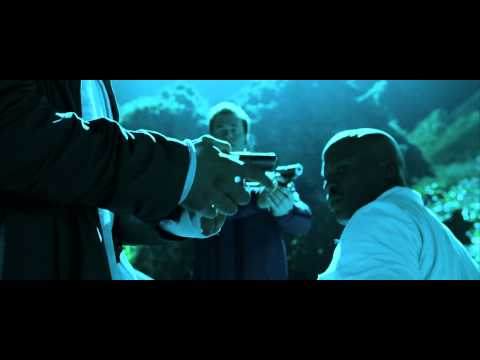Anonymous
Anonymous asked in Science & MathematicsMathematics · 3 months ago

# Are 3/5 good odds?

Is it like 75%?

Relevance

No, because 3/5 as a percentage is 60%

•Login to reply the answers
• 3/5 equals 60%. Whether those are good odds, depends on the situation.

Chances of winning in a casino: good odds.

Chances of the bullet still being in the revolver with Russian roulette, not such good odds.

•Login to reply the answers
• 3/5 is like 60%.

•Login to reply the answers
• There is a lot of confusion about the difference between "odds" and "probability". Even though the terms are sometimes used interchangeably, they refer to different things.

You reference 3/5 as "odds". In that format it more correctly refers to probability. If you mean the probability of success is 3/5, that is  60% which is more than 50% and might be considered a favorable state of affairs in which you could expect to win 3 times out of every 5 times you try. In terms of "odds", a percentage of 3/5 would be expressed at 3 to 2.

If you mean to refer to 3/5 as "odds", they are more correctly stated as 3 to 5. That means that for every 3 wins, you can expect 5 losses. The converts to 3 successes out of 3+5 = 8 trials or a percentage of 3/8 = 37.5%.

So odds, expressed at 3 to 5 means of every 8 trials, you win 3 and lose 5. Those are not very good odds.

Odds expressed as A to B convert to a percentage of A/(A+B)

A percentage expressed as A/B converts to odds of A to B-A

•Login to reply the answers
• NO!!! it is more like 60%

3/5 = 0.6 = 60%

•Login to reply the answers
• 3/5 x 100 = 60%.

Reasonmable odds but could be a lot better

•Login to reply the answers
• 3/5 = 60/100 = 60% 60% is not great but is better than 50%

•Login to reply the answers
• 3/5 is 60%

3 to 5 is 37.5%

75% is 3/4 or 3 to 1•Login to reply the answers
•Login to reply the answers
•Login to reply the answers